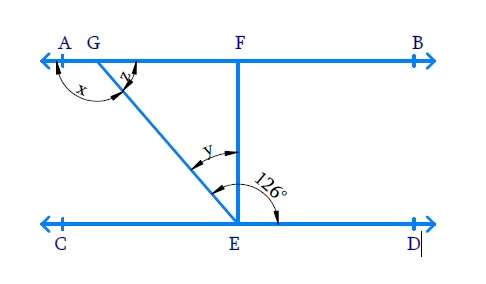# Ex.6.2 Q3 Lines and Angles Solution - NCERT Maths Class 9

Go back to  'Ex.6.2'

## Question

In the given figure, if $$AB \,‖\, CD$$, $$EF \,\bot \,CD$$ and $$\angle GED = 126^ {\circ},$$ find $$\angle AGE, \; \angle GEF$$ and $$\angle FGE.$$Video Solution
Lines And Angles
Ex 6.2 | Question 3

## Text Solution

What is known?

$$AB ‖ CD$$, $$\text{EF} \bot \text{CD}$$ and $$\angle GED = 126^ {\circ},$$

What is unknown?

$$\angle AGE =?,\;\; \angle GEF=?$$ and $$\angle FGE =?$$

Reasoning:

• When two lines intersect, adjacent angles formed are supplementary.
• When two parallel lines are cut by a transversal, alternate interior angles formed are equal.

Steps:

Let $$\angle AGE = x,\;\; \angle GED= y$$ and $$\angle FGE = z$$.

From the figure, we can see that,

\begin{align} \angle GED& = \angle GEF + \angle FED \\ y & = (126 ^ { \circ } - 90 ^ { \circ }) \\ \angle GEF & = y = 36 ^ { \circ } \end{align}

$$AB$$ and $$CD$$ are parallel lines cut by a transversal, the pair of alternative angles formed are equal.

\begin{align} \angle AGE &= \angle GED \\ \angle AGE &= x = 126 ^ { \circ } \end{align}

Line $$AB$$ is intersected by line $$GE$$ hence adjacent angles formed are supplementary.

\begin{align} x + z &= 180 ^ { \circ } \\ 126 ^ { \circ } + z &= 180 ^ { \circ } \\ z &= 180 ^ { \circ } - 126 ^ {\circ } \\ \quad &= 54 ^ {\circ} \\ \angle FGE = z &= 54 ^ { \circ } \end{align}

Video Solution
Lines And Angles
Ex 6.2 | Question 3

Learn from the best math teachers and top your exams

• Live one on one classroom and doubt clearing
• Practice worksheets in and after class for conceptual clarity
• Personalized curriculum to keep up with school